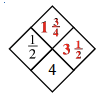### Home > CC3 > Chapter 1 > Lesson 1.1.2 > Problem1-15

1-15.

Recall the Diamond Problem pattern that you found in problem 1-4, which is represented in the diamond at right. Copy and complete the Diamond Problems below using the same pattern.1.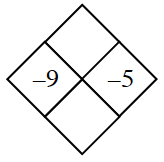Multiply the two given numbers together to get the top number. Add the given numbers together to get the bottom number.

The product of two negative numbers is positive. The sum of two negative numbers is negative.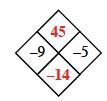1.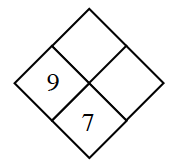What added to $9$ equals $7$?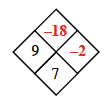1.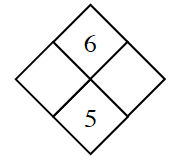What are the factors of $6$? Which pair has a sum of $5$?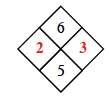1.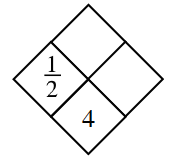Subtract one half from $4$ to find the right-side number.